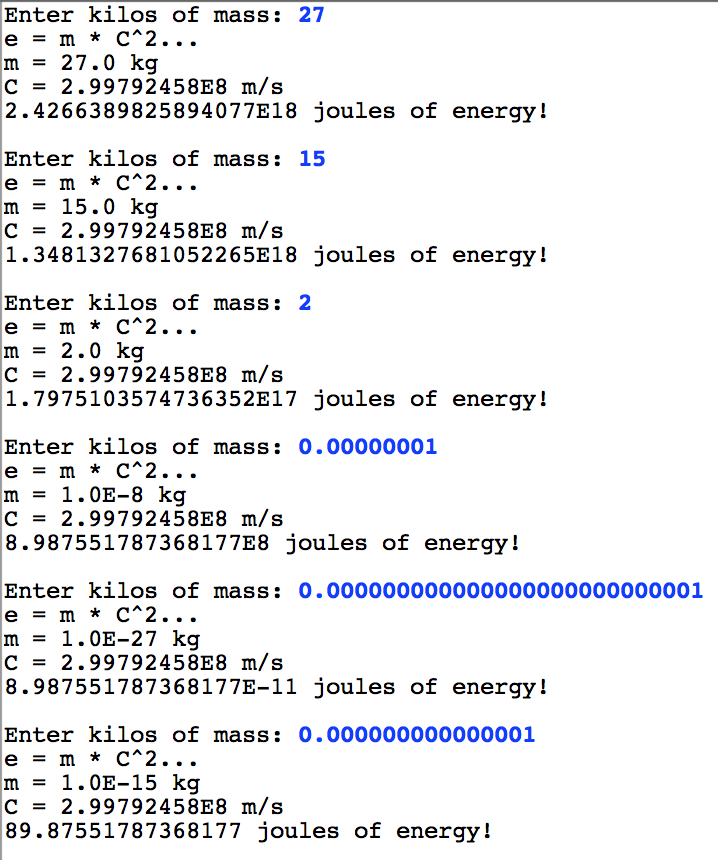One of the exciting human discoveries is that mass and energy are interchangable and are related by the equation E = M × C 2. This relationship was uncovered by Albert Einstein almost 100 years ago and we now know that the sun produces its heat by transforming small amounts of mass into large amounts of energy.

Write a program that continually reads in mass from the user and then outputs the amount of energy. Show your work in the following way:If you ask the user to input kilograms, and you use speed of light equals 299792458 (which is in meters per second) the result of calculating E = M × C 2 will be in Jouls.

Note that 3.0E8 is scientific notation for 3.0 × 10 8... which is 300,000,000. You don't need to do anything for java to print doubles in scientific notation. It will automatically do so when numbers get big enough.

Since the speed of light will never change, it makes sense to declare it as a constant.

## Solution

```/**
* Program: EMC2
* -------------
* This program helps users calculate how much energy they could
* get if they transformed their mass. Thanks Einstein!
*/
public class EMC2 extends ConsoleProgram {

// This declares a constant... it can never change value.
// Speed of light in m/s
private static final double C = 299792458;

public void run() {
// Repeats forever
while(true) {
// Read the mass in from the user.
double massInKg = readDouble("Enter kilos of mass: ");

// Calculate energy
double energyInJoules = massInKg * C * C;

// Display work to the user
println("e = m * C^2...");
println("m = " + massInKg + " kg");
println("C = " + C + " m/s");
println(energyInJoules + " joules of energy!");
println("");
}
}
}```